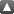Items where Author is "Bégout, Pascal"Up a level
 Export as ASCII CitationBibTeXDublin CoreEP3 XMLEndNoteHTML CitationJSONMETSMultiline CSVObject IDsOpenURL ContextObjectRDF+N-TriplesRDF+N3RDF+XMLRefWorksReferReference Manager
Group by: Item Type | Date | No Grouping
Number of items: 16.

Article

Bégout, Pascal, Bolte, Jérôme and Jendoubi, Mohamed Ali (2015) On damped second-order gradient systems. Journal of Differential Equations, vol. 259 (n° 7). pp. 3115-3143.

Bégout, Pascal and Diaz, Jesus Ildefonso (2015) Existence of weak solutions to some stationary Schrödinger equations with singular nonlinearity. Revista de la Real Academia de Ciencias Exactas, Fisicas y Naturales. Serie A. Matematicas, 109 (1). pp. 43-63.

Bégout, Pascal and Diaz, Jesus Ildefonso (2014) Self-similar solutions with compactly supported profile of some nonlinear Schrödinger equations. Electronic Journal of Differential Equations, vol. 90. pp. 1-15.

Bégout, Pascal and Diaz, Jesus Ildefonso (2014) A sharper energy method for the localization of the support to some stationary Schrodinger equations with a singular nonlinearity. Discrete and Continuous Dynamical Systems, vol. 34 (n° 9). pp. 3371-3382.

Bégout, Pascal and Diaz, Jesus Ildefonso (2012) Localizing Estimates of the Support of Solutions of some Nonlinear Schrödinger Equations - The Stationary Case. Annales de l'Institut Henri Poincaré. Analyse Non Linéaire, vol. 29 (n° 1). pp. 35-58.

Bégout, Pascal and Soria, Fernando (2007) A generalized interpolation inequality and its application to the stabilization of damped equations. Journal of Differential Equations, vol. 240 (n° 2). pp. 324-356.

Bégout, Pascal and Vargas, Ana (2007) Mass Concentration Phenomena for the L2-Critical Nonlinear Schrödinger Equation. Transactions of the American Mathematical Society, vol. 359 (n° 11). pp. 5257-5282.

Bégout, Pascal and Vargas, Ana (2007) Mass concentration for the L²-critical nonlinear Schrödinger equation. Transactions of the American Mathematical Society, vol. 359 (n° 11). pp. 5257-5282.

Bégout, Pascal and Diaz, Jesus Ildefonso (2006) On a nonlinear Schrödinger equation with a localizing effect. Comptes Rendus Mathématique. Académie des Sciences, vol. 342 (n° 7). pp. 459-463.

Bégout, Pascal (2004) Maximum decay rate for finite-energy solutions of nonlinear Schrödinger equations. Differential and Integral Equations. An International Journal for Theory & Applications, vol. 17 (n° 11). pp. 1411-1422.

Bégout, Pascal (2004) Maximum decay rate for the nonlinear Schrödinger equation. Nonlinear Differential Equations And Applications, vol. 11 (n° 4). pp. 451-467.

Bégout, Pascal (2002) Necessary Conditions and Sufficient Conditions for Global Existence in the Nonlinear Schrödinger Equation. Advances in Mathematical Sciences and Applications, 12 (2). pp. 817-827.

Bégout, Pascal (2002) Necessary conditions and sufficient conditions for global existence in the nonlinear Schrödinger equation. Advances in Mathematical Sciences and Applications, vol. 12 (n° 2). pp. 817-827.

Bégout, Pascal (2001) Convergence to scattering states in the nonlinear Schrödinger equation. Communications in Contemporary Mathematics (ccm), vol. 3 (n° 3). pp. 403-418.

Monograph

Bégout, Pascal and Diaz, Jesus Ildefonso (2013) Self-similar solutions with compactly supported profile of some nonlinear Schrödinger equations. TSE Working Paper, n. 13-400

Bégout, Pascal and Diaz, Jesus Ildefonso (2013) A sharper energy method for the localization of the support to some stationary Schrödinger equations with a singular nonlinearity. TSE Working Paper, n. 13-401

This list was generated on Mon Oct 21 00:51:45 2019 CEST.